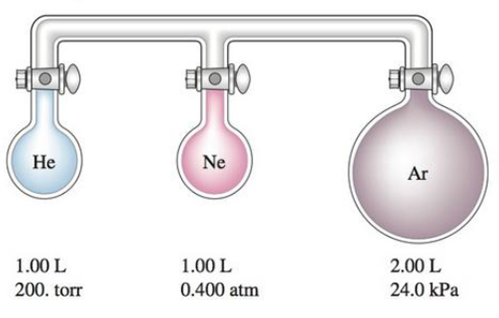# Consider the three flasks in the diagram below. Assuming the connecting tubes have negligible volume, what is the partial pressure of each gas and the total pressure after all the stopcocks are opened?### Chemistry: An Atoms First Approach

2nd Edition
Steven S. Zumdahl + 1 other
Publisher: Cengage Learning
ISBN: 9781305079243

#### Solutions

Chapter
Section### Chemistry: An Atoms First Approach

2nd Edition
Steven S. Zumdahl + 1 other
Publisher: Cengage Learning
ISBN: 9781305079243
Chapter 8, Problem 87E
Textbook Problem
6370 views

## Consider the three flasks in the diagram below. Assuming the connecting tubes have negligible volume, what is the partial pressure of each gas and the total pressure after all the stopcocks are opened?Interpretation Introduction

Interpretation: The total pressure, the partial pressures of each gas in the given figure is needed to be determined when the stop-cocks are opened.

Concept introduction:

• Partial pressure of a gas in a mixture of gases is the pressure of that gas when it alone.
• Total pressure of a mixture of gases is the sum of individual partial pressures of constituted gases.
• According to Boyle’s law of gas molecules,

If the volume of gases in a container is increased then the pressure will decrease at constant temperature and number of moles.

Equation for Boyle’s law is,

PinitialVinitial=PfinalVfinal

### Explanation of Solution

Explanation

In the figure,

In initial condition, the stop-cocks of each container are closed.

In final condition, the stop-cocks of each container are opened.

Therefore,

The initial pressure of He gas is 200torr

The initial volume of He gas is 1L

The final volume is the sum of volumes of three containers =1L+1L+2L=4L

The partial pressure of He is the final pressure in according to Boyle’s law.

Equation for Boyle’s law states,

PinitialVinitial=PfinalVfinal

Therefore,

Partial pressure of He is,

Pfinal=200torr×1L4L =50torr

Hence, the partial pressure of He when the stop-cocks are opened in the figure is 50torr .

In the figure,

In initial condition, the stop-cocks of each container are closed.

In final condition, the stop-cocks of each container are opened.

Therefore,

The initial pressure of Ne gas is 0.4atm=0.4atm×760torr1atm=304torr

The initial volume of Ne gas is 1L

The final volume is the sum of volumes of three containers =1L+1L+2L=4L

The partial pressure of Ne is the final pressure in according to Boyle’s law.

Equation for Boyle’s law states,

PinitialVinitial=PfinalVfinal

Therefore,

Partial pressure of He is,

Pfinal=304torr×1L4L =76torr

Hence, the partial pressure of Ne when the stop-cocks are opened in the figure is 76torr

### Still sussing out bartleby?

Check out a sample textbook solution.

See a sample solution

#### The Solution to Your Study Problems

Bartleby provides explanations to thousands of textbook problems written by our experts, many with advanced degrees!

Get Started

Find more solutions based on key concepts
Most people who successfully maintain weight loss do all of the following except continue to employ many of the...

Nutrition: Concepts and Controversies - Standalone book (MindTap Course List)

What is the difference between artificial selection and natural selection?

Biology: The Dynamic Science (MindTap Course List)

What are the three most common chronic conditions affecting men over age 65?

Nutrition Through the Life Cycle (MindTap Course List)

You attach an object to the bottom end of a hanging vertical spring. It hangs at rest alter extending the sprin...

Physics for Scientists and Engineers, Technology Update (No access codes included)

Hydrocarbons, saturated, unsaturated.

Introductory Chemistry: An Active Learning Approach

Why is water a polar molecule (review Chapter 6)?

Oceanography: An Invitation To Marine Science, Loose-leaf Versin